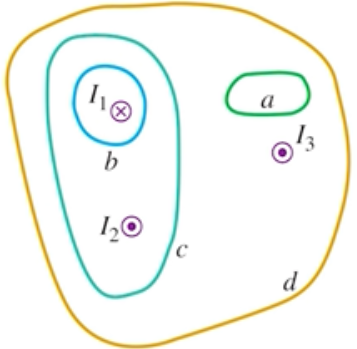Need Help?

Subscribe to Physics 2

###### \${selected_topic_name}
• Notes

$\begin{array}{l}{\text { Figure } \mathrm{E} 28.42 \text { shows, in }} \\ {\text { cross section, several conductors }} \\ {\text { that carry currents through the }} \\ {\text { plane of the figure. The currents }} \\ {\text { have the magnitudes } I_{1}=4.0 \mathrm{A}} \\ {I_{2}=6.0 \mathrm{A}, \text { and } I_{3}=2.0 \mathrm{A}, \text { and }}\end{array}$

$\begin{array}{l}{\text { the directions shown. Four paths, }} \\ {\text { labeled a through } d \text { , are shown. }} \\ {\text { What is the line integral } \oint \vec{B} \cdot d \vec{l}} \\ {\text { for each path? Each integral in- }} \\ {\text { volves going around the path in }} \\ {\text { the counterclockwise direction. Ex- }} \\ {\text { plain your answers. }}\end{array}$$\oint \vec{B} \cdot \vec{d}=M_{0} I_\text { encl }$

(a) $I_{\text {encl }}=0$$\longrightarrow \oint \vec{B} \cdot d \vec{l}=0$

(b) $I_\text { encl }=-I_{1}$$=-4 A \longrightarrow \oint \vec{B} \cdot d \vec{L}$$=M_{0}(-4)$$=-5.03 * 10^{-6} \mathrm{T.m}$

(c) $I_\text { encl }=-I_{1}+I_{2}=$$-4+6=2 A$$\rightarrow \oint \vec{B} \cdot \vec{d} L$$=M_{0}(2)=2.51*10^{-6} \mathrm{T.m}$

(d) $I_{encl} d=$$-I_{1}+I_{2}+I_{3} =$$-4+6+2=4 A \rightarrow \oint \vec{B} d \vec{L}=M_{0}(4)$

$∴ \quad=5.03 * 10^{-6} \mathrm{T.m}$

$\begin{array}{l}{\text { A closed curve encircles several conductors. The line }} \\ {\text { integral } \oint \vec{B} \cdot d \vec{l} \text { around this curve is } 3.83 \times 10^{-4} \mathrm{T} \cdot \mathrm{m} . \text { (a) What }} \\ {\text { is the net current in the conductors? (b) If you were to integrate }} \\ {\text { around the curve in the opposite direction, what would be the value }} \\ {\text { of the line integral? Explain. }}\end{array}$

$\oint \vec{B} \cdot d \vec{l}$$=M_0 I _{encl}$

(a) $\oint \vec B \cdot d \vec{l}$$=M_{0} I_{encl}$

$3.83 * 10^{-4}=4 \pi * 10^{-7} I_\text {encl } \longrightarrow I_\text { encl }=305 \mathrm{A}$

(b) $-3.83 * 10^{-4} \mathrm{T} \cdot \mathrm{m}$